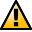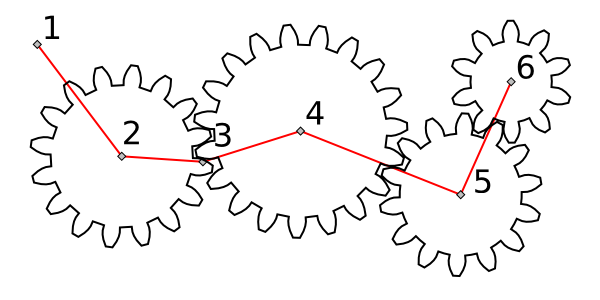WarningThis section not yet updated for Inkscape v0.92.

## Gears

This effect draws a series of intermeshed gears. It is more of a toy effect, designed to demonstrate the possibilities of LPEs. The Gear extension can also be used to draw gears with a bit more control.

The effect uses the nodes of a path to determine how the gears are drawn. At least three nodes are needed to specify the first gear. Additional gears require one additional node each. Some nodes may be skipped if they would result in impractical gears.An example of using the Gear LPE. The original path is shown in red. Point 1 defines the orientation of the first gear. Point 2 defines the center of the first gear. Point 3 defines the radius of the first gear. Points 4, 5, and 6 determine the centers of additional gears. The radius of the second gear is determined by subtracting the radius of the first gear from the distance between the centers of the first and second gears.

Two parameters are available: Teeth determines the number of teeth on the first gear. Phi determines the Pressure Angle of the gears. For real gears the Pressure Angle is typically 14.5, 20, or 25 degrees. Note: The default angle is 5 degrees, not a very realistic value.